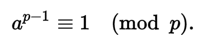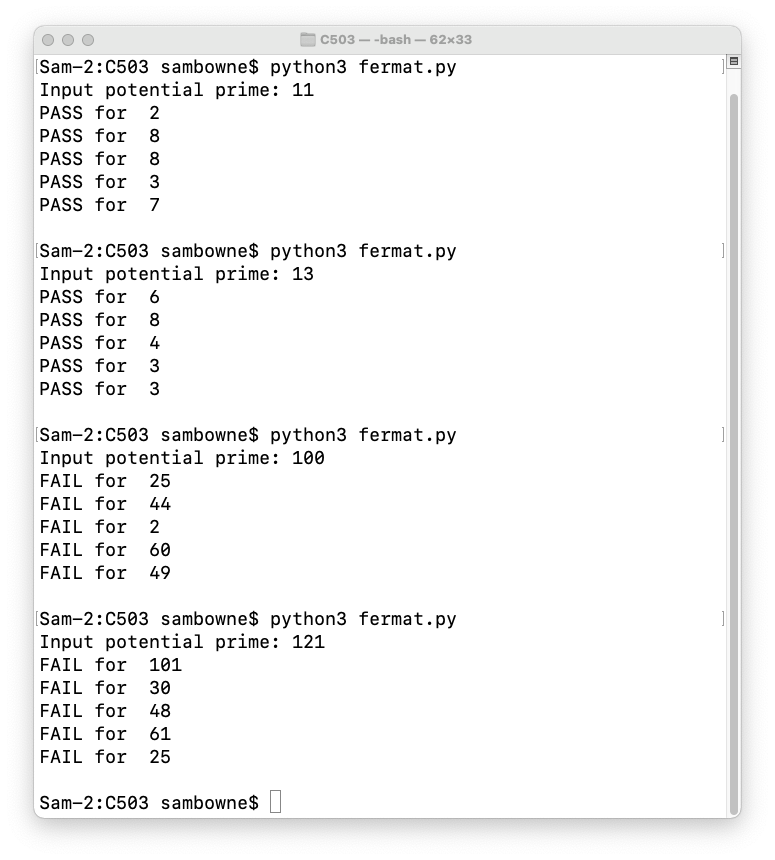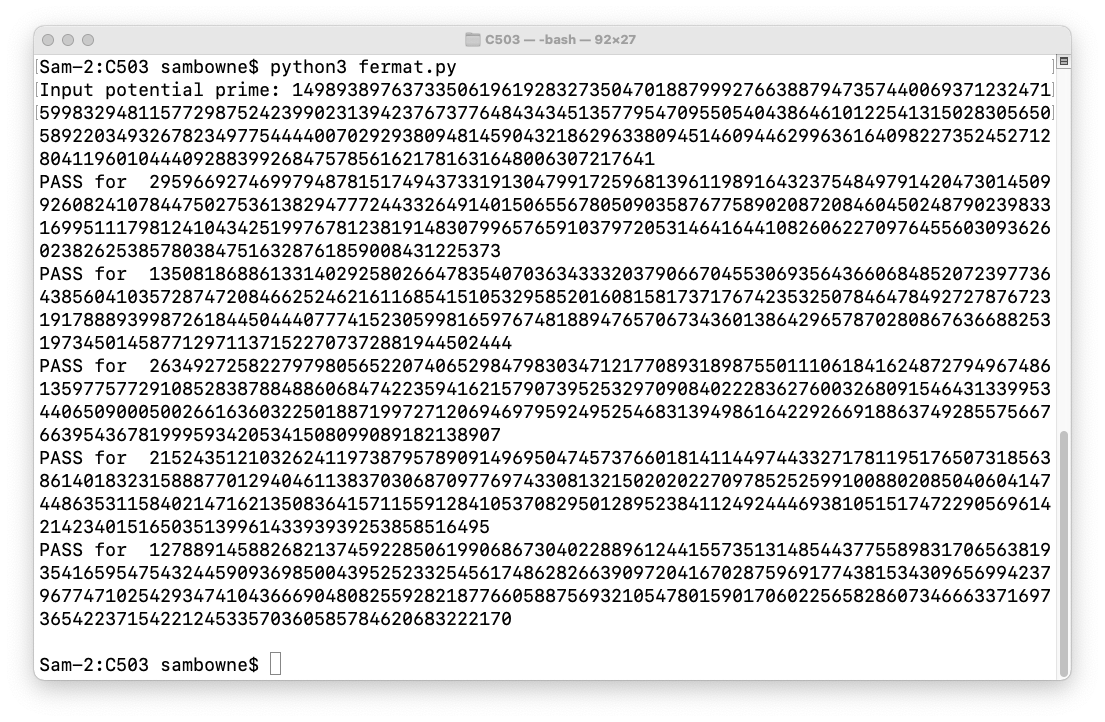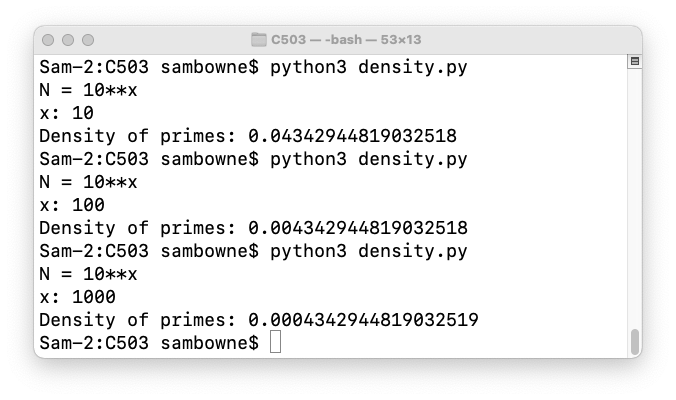C 503: Finding Large Primes

What you need:
• Any computer with Python 3.

Purpose

To implement the Fermat primality test and find large primes.

Background

Fermat's little theorem states that if p is prime and a is not divisible by p, then:So testing that condition for several random values of a can be used as evidence that p is probably prime.

This site explains the Fermat primality test and its flaws in more detail:

Simple Python Implementation

Create a file named fermat.py containing this code:
from random import randint

p = int(input("Input potential prime: "))

for i in range(5):
a = randint(2,p-2)
if pow(a, p-1, p) == 1:
print("PASS for ", a)
else:
print("FAIL for ", a)
print()
Run the file with this command:
python3 fermat.py

Demonstration

Try these values: 11, 13, 100, 121.

As shown below, 11 and 13 pass all the tests because they are prime, but 100 and 121 fail because they aren't.Testing Large Numbers

Test this number, which is 1024 bits long, and is prime:

149893897637335061961928327350470188799927663887947357440069371232471599832948115772987524239902313942376737764843434513577954709550540438646101225413150283056505892203493267823497754444007029293809481459043218629633809451460944629963616409822735245271280411960104440928839926847578561621781631648006307217641

It works, because Python really can handle any size of integer, as shown below.Density of Primes

How many guesses will it take to find a prime number? The prime number theorem says the probability of a number less than N being prime is:
1 / ln(N)
Or, in Python:
import math

print("N = 10**x")
x = int(input("x: "))
n = 10**x
print("Density of primes:", 1/math.log(n))
As shown below, even for the immense number 10**1000, primes are still pretty common, about 4 in 10,000.C 503.1: Find the Next Prime (10 pts)

This number is not prime:

10**300

Find the first number above it which is prime.

The flag is that number as one long integer, like

11111111111111111111111111111111

C 503.2: Companion Primes (10 pts extra)

11 and 13 are both primes, only differing by two. Such primes are called companion primes.

Find the first set of companion primes above

10**100

The flag is the lower of the two primes, as one long integer, like

11111111111111111111111111111111

Posted 11-1-17 by Sam Bowne
Added to Crypto Hero 4-16-18 6:26 am
Ported to new scoring engine 7-8-19
Updated to python 3 11-5-20